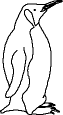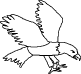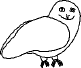EnchantedLearning.com is a user-supported site.
As a bonus, site members have access to a banner-ad-free version of the site, with print-friendly pages.

 You might also like: Divide Ten Pets Worksheet Printout Divide Six by 2, 3, and 6 Worksheet Printout Divide Nine Flowers Worksheet Printout Divide Twelve Farm Animals Worksheet Printout Divide Fruit into Four Groups Worksheet Printout Today's featured page: Plant Cell Label Me! Glossary

 Go to the AnswersMath Pages EnchantedLearning.com Divide Eight Birds Divide the birds into groups to do division. Visual Division PagesDivision Pages

 Divide the penguins into two equal groups.How many penguins are there? How many groups of penguins are there? How many penguins are in each group?
8 ÷ 2 =

 Divide the eagles into four equal groups.How many eagles are there? How many groups of eagles are there? How many eagles are in each group?
8 ÷ 4 =

 Divide the owls into eight equal groups.How many owls are there? How many groups of owls are there? How many owls are in each group?
8 ÷ 8 =

## Enchanted Learning Search

 Search the Enchanted Learning website for: